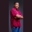Related Tags

c#
communitycreator

# How to calculate the area of a sphere in C#Theodore Kelechukwu Onyejiaku

## Overview

The area of a sphere is the total area covered by the sphere's outer surface. In C#, we can calculate it using the sphere's radius.

### Syntax

4 * Math.PI * r * r
Syntax for calculating area of a Sphere in C#

### Parameters

• Math.PI: This is an built-in value that represents the mathematical value Pi.
• r: This represents the radius of the sphere.

### Example

using System;
class HelloWorld
{
// create function to get the areas
static void GetArea(double r){
double area = 4 * Math.PI * r * r;
Console.WriteLine("The area of the sphere with radius "+r+" is "+area);
}

// main method
static void Main()
{
// create radius of some spheres
double r1 = 10;
double r2 = 1.5;
double r3 = 4.5;
double r4 = 8;

// get the area of the spheres
GetArea(r1);
GetArea(r2);
GetArea(r3);
GetArea(r4);
}
}
Get the area of a Sphere in C#

### Explanation

• Line 5: We create a method called GetArea(). It takes the sphere's radius, calculates the area, then prints the result to the console screen.
• Lines 14–17: In the Main method, we create the radii of some spheres.
• Lines 20–23: We call the GetArea() method, and display the results on the console.

RELATED TAGS

c#
communitycreator

CONTRIBUTORTheodore Kelechukwu Onyejiaku
RELATED COURSES

View all Courses

Keep Exploring

Learn in-demand tech skills in half the time